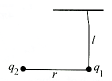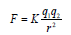# Electrostatic MCQ Level - 1

## 30 Questions MCQ Test Exclusive Video Lectures of Class 12 Physics by Experts | Electrostatic MCQ Level - 1

Description
This mock test of Electrostatic MCQ Level - 1 for Class 12 helps you for every Class 12 entrance exam. This contains 30 Multiple Choice Questions for Class 12 Electrostatic MCQ Level - 1 (mcq) to study with solutions a complete question bank. The solved questions answers in this Electrostatic MCQ Level - 1 quiz give you a good mix of easy questions and tough questions. Class 12 students definitely take this Electrostatic MCQ Level - 1 exercise for a better result in the exam. You can find other Electrostatic MCQ Level - 1 extra questions, long questions & short questions for Class 12 on EduRev as well by searching above.
QUESTION: 1

Solution:
QUESTION: 2

Solution:
QUESTION: 3

### If a body is charged by rubbing it, its weight

Solution:
QUESTION: 4

In S.I system, the value of e0 is

Solution:
QUESTION: 5

Dimensions of e0 are

Solution:
QUESTION: 6

The dielectric constant K of an insulator can be :

Solution:
QUESTION: 7

Choose the correct statement :

Solution:
QUESTION: 8

There are two charges +1 μC and +5 μC. The ratio ofthe forces (force on one due to other) acting on themwill be

Solution:
QUESTION: 9

Five balls numbered 1, 2, 3, 4, 5 are suspended usingseparate threads. The balls (1, 2), (2, 4) and (4, 1) showelectrostatic attraction, while balls (2,3) and (4, 5) showrepulsion, Therefore, ball 1 must be

Solution:
QUESTION: 10

Electric charges A and B repel each other, Electriccharges B and C also repel other. If A and C are heldclose together, they will.

Solution:
QUESTION: 11

Two point charges repel each other with a force of 100N One of the charges is increased by 10% and theother is reduced by 10%. The new force of repulsion atthe same distance would be

Solution:
QUESTION: 12

Two positive and equal charges are fixed at a certaindistance. A third small charge is placed in between thetwo charges and it experiences zero net force due tothe other two.

Solution:
QUESTION: 13

An isolated charge q1 of mass m is suspended freelyby a thread of lengthi . Another charge q2 is broughtnear it (r >>i ). When q1 is in equilibrium, tension inthread will beSolution:
QUESTION: 14

A point charge q1, is moved along a circular path ofradius r in the electric field of another point charge q2 at the centre of the parh. The work done by the electric field on the charge q1 in half revolution is

Solution:
QUESTION: 15

A spherical conducting ball is suspended by a groundedconducting thread. A positive point charge is moved nearthe ball. The ball will

Solution:
QUESTION: 16

Five point charges, each of value +q, are placed on fivevertices of a regular hexagon of side L. The magnitudeof the force on a point charge of value -q coulomb placedthe centre of the hexagon is

Solution:
QUESTION: 17

The sure test of electrification is :

Solution:
QUESTION: 18

The number of electrons in one coulomb of charge willbe :

Solution:
QUESTION: 19

The minimum value of charge on any charged body maybe :

Solution:
QUESTION: 20

When 1014 electrons are removed from a neutral metalsphere, the charge on the sphere becomes

Solution:
QUESTION: 21

One metallic sphere A is given a positive charge whereasanother metallic sphere B of exactly the same mass isgiven a negative charge. Then

Solution:
QUESTION: 22

An electron has charge 1.6 × 10-19 coulomb when atrest. When it moves with speed 0.8c the charge on theelectron would be (c being speed of light in vacuum)

Solution:
QUESTION: 23

A charged rod attracts bits of dry cork dust which aftertouching the rod

Solution:
QUESTION: 24

The electrostatic force between two point charges q1and q2 at separation r is given byThe constant K

Solution:
QUESTION: 25

A charge q1 exerts some force on a second charge q2.If third charge q3 is brought near, the force of q1 exertedon q2

Solution:
QUESTION: 26

If Fe and Fg are electric and gravitational force,respectively between two electrons situated at certaindistance apart, then the ratio of Fe and Fg is of the order of

Solution:
QUESTION: 27

A thin stream of water from a tap is attracted by placing a rod near it. The rod is

Solution:
QUESTION: 28

The proton is 1836 times heavier than electron. TheCoulomb's force of repulsion between two protons at acertain distance between them is F. Then the Coulombforce between two electrons for same distance wouldbe :

Solution:
QUESTION: 29

Two point charges at a distance r air exerts a force on each other The value of distance R at which they experience the same force when placed in a medium of dielectric constant is?

Solution:
QUESTION: 30

Two neutrons are placed at some distance a part from each other. They will

Solution:

However, there is a concept in quantum mechanics which is beyond the scope of grade XII. This concept is Pauli's Exclusion principle. Neutrons are spin 1/2 particles. According to Pauli's exclusion principle, two identical spin particles cannot occupy the same state. Hence, a strong repulsive force will be generated between the two neutrons. So, they will repel each other.